# refline

## Syntax

``refline(m,b)``
``refline(coeffs)``
``refline``
``refline(ax,___)``
``hline = refline(___)``

## Description

````refline(m,b)` adds a reference line with slope `m` and intercept `b` to the current axes.```

example

````refline(coeffs)` adds the line defined by the elements of the vector `coeffs` to the figure.```

example

``refline` with no input arguments is equivalent to `lsline`.`

example

````refline(ax,___)` adds a reference line to the plot in the axis specified by `ax`, using any of the input arguments in the previous syntaxes.```

example

````hline = refline(___)` returns the reference line object `hline` using any of the input arguments in the previous syntaxes. Use `hline` to modify properties of a specific reference line after you create it. For a list of properties, see Line Properties.```

## Examples

collapse all

Generate sample data for an independent variable `x` and a dependent variable `y` .

```x = 1:10; y = x + randn(1,10);```

Create a scatter plot of `x` and `y` .

`scatter(x,y,25,'b','*')`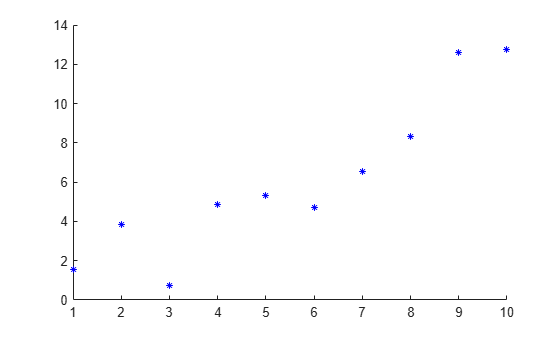Superimpose a least-squares line on the scatter plot.

`refline`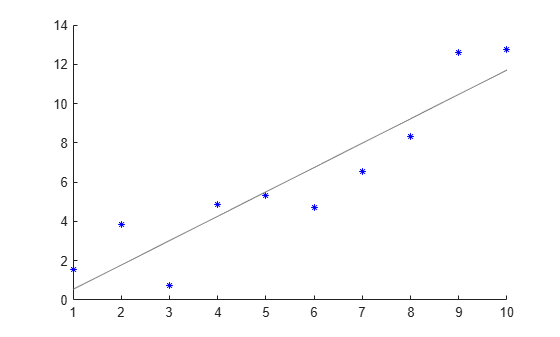Add a reference line at the mean of the scatter plot.

```mu = mean(y); hline = refline([0 mu]); hline.Color = 'r';```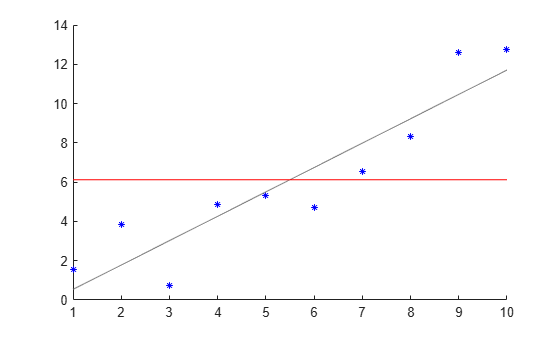The red line is the reference line at the mean of the data.

Define the x-variable and two different y-variables to use for the plots.

```rng default % For reproducibility x = 1:10; y1 = x + randn(1,10); y2 = 2*x + randn(1,10);```

Define `ax1` as the top half of the figure, and `ax2` as the bottom half of the figure. Create the first scatter plot on the top axis using `y1`, and the second scatter plot on the bottom axis using `y2`.

```figure ax1 = subplot(2,1,1); ax2 = subplot(2,1,2); scatter(ax1,x,y1) scatter(ax2,x,y2)```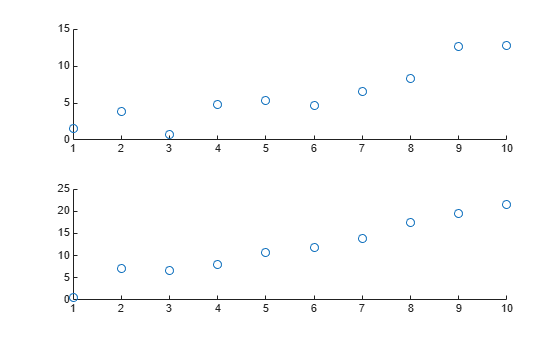Superimpose a least-squares line on the top plot, and a reference line at the mean of the `y2` values in the bottom plot.

```lsline(ax1) % This is equivalent to refline(ax1) mu = mean(y2); refline(ax2,[0 mu])```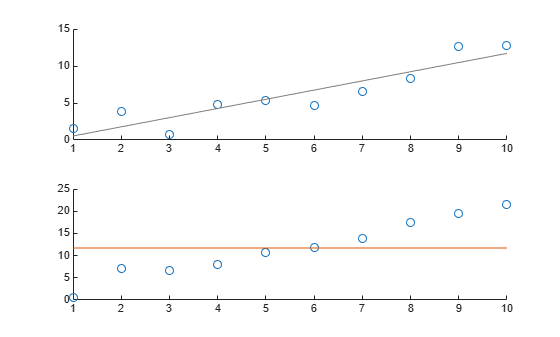## Input Arguments

collapse all

Slope of the reference line, specified as a numeric scalar. The function uses `m` to define the line

` y = m*x + b. `

Example: `refline(-1,1)`

Data Types: `single` | `double`

Intercept of the reference line, specified as a numeric scalar. The function uses `b` to define the line

` y = m*x + b. `

Example: `refline(2,-10)`

Data Types: `single` | `double`

Linear coefficients, specified as a length-two numeric vector. `coeffs` contains the coefficients of a line defined as

` y = coeffs(1)*x + coeffs(2). `

Example: `refline([-1,2])`

Data Types: `single` | `double`

Target axes, specified as an `axes` object. If you do not specify the axes and if the current axes are Cartesian axes, then the `refline` function uses the current axes.

## Output Arguments

collapse all

One or more reference line objects, returned as a scalar or a vector. These objects are unique identifiers, which you can use to query and modify properties of a specific reference line. For a list of properties, see Line Properties.